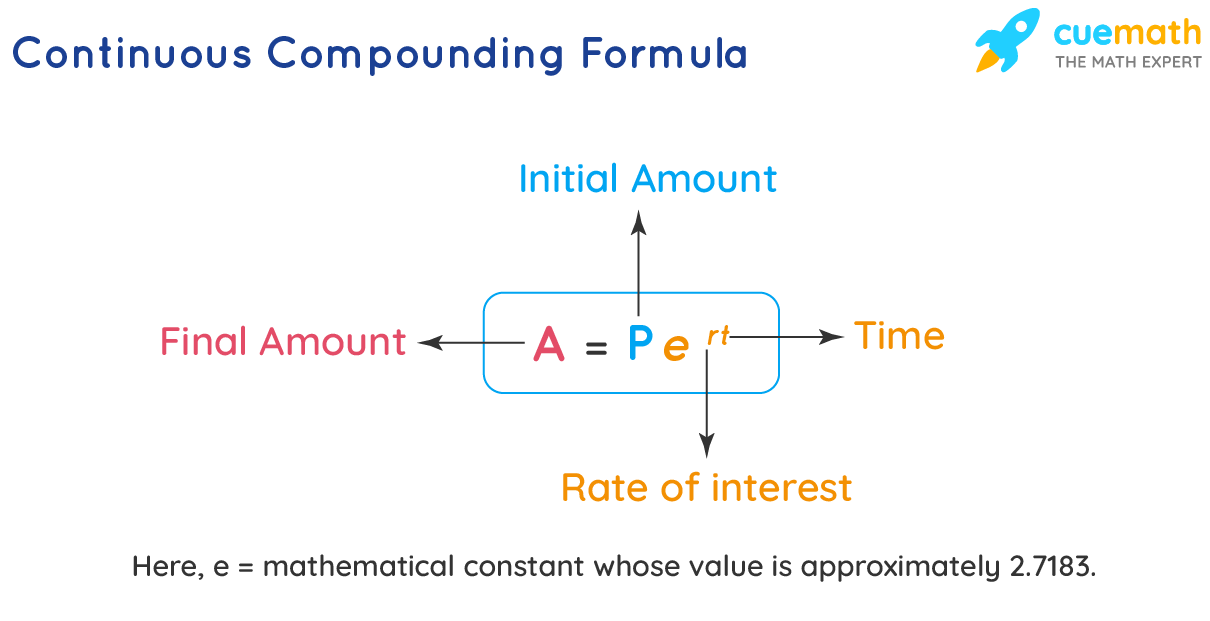# Continuous Compounding Formula

Compound interest is usually calculated on a daily, weekly, monthly, quarterly, half-yearly, or annual basis. In each of these cases, the number of times it is compounding is different and is finite. But what if this number is infinite? This leads to the continuous compounding formula. In continuous compounding number of times by which compounding occurs is tending to infinity. Let us learn the continuous compounding formula along with a few solved examples.

## What is Continuous Compounding Formula?

The compound interest formula is,

A = P (1 + r/n)nt

Here, n = the number of terms the initial amount (P) is compounding in the time t.

A is the final amount (or) future value.

For the continuous compound interest, n → ∞. So we will take the limit of the above formula as n → ∞.

A = lim$$_{n \rightarrow \infty}$$ P (1 + r/n)nt = Pert  (∵ lim$$_{n \rightarrow \infty}$$ (1 + r/n)= er)

Thus, the continuous compound interest formula is,

A = Pert## Solved Examples Using Continuous Compounding Formula

### Tina invested $3000 in a bank that pays an annual interest rate of 7% compounded continuously. What is the amount she can get after 5 years from the bank? Round your answer to the nearest integer. Solution To find: The amount after 5 years. The initial amount is P =$3000.

The interest rate is, r = 7% = 7/100 = 0.07.

Time is, t = 5 years.

Substitute these values in the continuous compounding formula,

A = Pert

A = 3000 × e0.07(5) ≈ 4257

The answer is calculated using the calculator and is rounded to the nearest integer.

Answer: The amount after 5 years = $4,257. ### Example 2 ### What should be the rate of interest for the amount of$5,300 to become double in 8 years if the amount is compounding continuously? Round your answer to the nearest tenths.

Solution

To find: The rate of interest, r.

The initial amount is, P = $5,300. The final amount is, A = 2(5300) =$10,600.

Time is, t = 8 years.

Substitute all these values in the continuous compound interest formula,

A = Pert

10600 = 5300 × er (8)

Dividing both sides by 5300,

2 = e8r

Taking "ln" on both sides,

ln 2 = 8r

Dividing both sides by 8,

r = (ln 2) / 8 ≈ 0.087 (using calculator)

So the rate of interest = 0.087 × 100 = 8.7

Answer: The rate of interest = 8.7%.

Learn from the best math teachers and top your exams

• Live one on one classroom and doubt clearing
• Practice worksheets in and after class for conceptual clarity
• Personalized curriculum to keep up with school